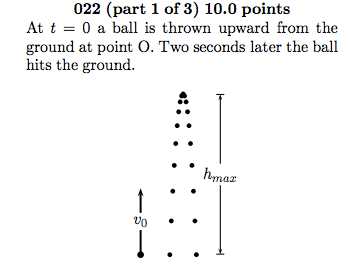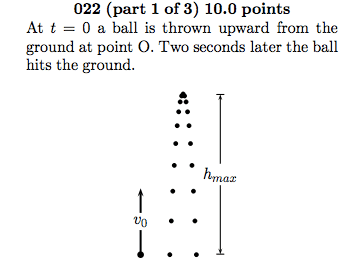# Finding velocity (having trouble interpreting the question

• zx636r
I know this answer is yahoo answers or something, but I refrained from just looking at the answer because I really want to understand what is going on.point O is the groundYou're shown a parabolic path/trajectory."O" is at ground level, or y = 0 describes a line "O" which you could regard as the "x-axis."A on its way up, where OA is 1/3Think "y = A."arbitrary point that is just deemed "A"?Call it an "arbitrary" altitude --- "A" for "arbitrary" or for "altitude" or for "ambiguous"f

## Homework Statement

For an initial velocity of V0, what is the velocity of the ball as it passes the point A on its way up, where OA is 1/3 the maximum height.

V0 = 10m/s
t = 1s (2s total for the ball to come back down)
maximum height = 5m
initial displacement x0 = 0m

## Homework Equations

Vf^2 = V0^2 + 2a(x - x0)

## The Attempt at a SolutionI'm assuming point O is the ground. If point OA is 1/3 of the maximum height then, it is 5/3m. My confusion is what point A should be. Is it some arbitrary point that is just deemed "A"? The answers are in terms of vA (velocity as the ball passes through point A) = initial velocity times a constant. The other thing I can think of is that I am wrong for setting point O at 0. Am I supposed to go another "O" distance from OA to get to point A?

I know this answer is yahoo answers or something, but I refrained from just looking at the answer because I really want to understand what is going on.

point O is the ground
You're shown a parabolic path/trajectory. "O" is at ground level, or y = 0 describes a line "O" which you could regard as the "x-axis."
A on its way up, where OA is 1/3
Think "y = A."
arbitrary point that is just deemed "A"?
Call it an "arbitrary" altitude --- "A" for "arbitrary" or for "altitude" or for "ambiguous" statement.

## Homework Statement

For an initial velocity of V0, what is the velocity of the ball as it passes the point A on its way up, where OA is 1/3 the maximum height.

V0 = 10m/s
t = 1s (2s total for the ball to come back down)
maximum height = 5m
initial displacement x0 = 0m

## Homework Equations

Vf^2 = V0^2 + 2a(x - x0)

## The Attempt at a SolutionI'm assuming point O is the ground.
Correct.
If point OA is 1/3 of the maximum height then, it is 5/3m. My confusion is what point A should be. Is it some arbitrary point that is just deemed "A"?
No. According to the information you gave it is at x = 5/3 m.

Is this supposed to be solved algebraically, or is the answer supposed to be for the specific problem of v0 = 10m/s, max ht = 5 m?

The answers are in terms of vA (velocity as the ball passes through point A) = initial velocity times a constant. The other thing I can think of is that I am wrong for setting point O at 0. Am I supposed to go another "O" distance from OA to get to point A?
No. These are both correct interpretations.

If you are trying to do this algebraically, then you use your equation to determine the maximum height x in terms of v0. To do that, you need to select the gravitational value of the acceleration a.

Chet